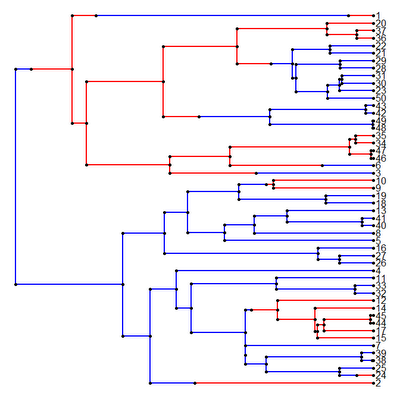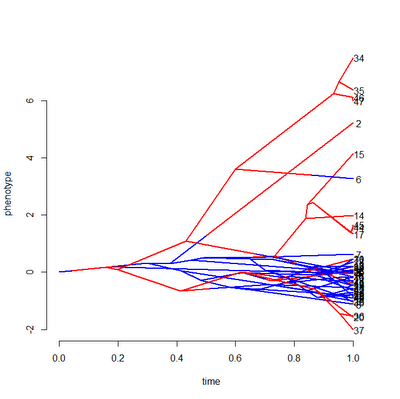## Tuesday, December 20, 2011

### Traitgram with mapped discrete character

After musing about doing so during my phytools phyloseminar (recorded here), I have just added the capacity to show a mapped discrete character trait to the new function phenogram. This could be a very neat way of, for instance, visualizing a change in the evolutionary rate or selection regime on some of the branches of the tree (although it will normally rely on ancestral character states estimated under a constant rate Brownian model).

The trick in doing this was not coloring the lines (that's trivial), but in mapping adjacent segments of the same branch. To do this, I first computed the slope of the entire branch (in this case, the change in phenotype over the change in the height above the root node), and then I used this slope, the length of the mapped segment, and the starting phenotypic trait value at the parent node to plot each line segment for each mapped state. Direct link to this new version of the function is here.

I'll illustrate the use (and utility) of the function by way of a simulated example:

> require(phytools); require(geiger)
> # simulate a tree of unit length
> tree<-rescaleTree(drop.tip(birthdeath.tree(b=1,d=0, taxa.stop=51),"51"),1)
> Q<-matrix(c(-1,1,1,-1),2,2)
> rownames(Q)<-colnames(Q)<-c("blue","red")
> # simulate discrete character history
> mtree<-sim.history(tree,Q,"blue")
> cols<-c("blue","red"); names(cols)<-cols
> plotSimmap(mtree,cols)> sig2<-c(1,10); names(sig2)<-cols
> # simulate rate depending on trait
> x<-sim.rates(mtree,sig2,internal=T)

And then we can plot the phenogram, including the mapped discrete character history:

> source("phenogram.R")
> phenogram(mtree,x,colors=cols)The traitgram, in this case, includes the known states at internal nodes and clearly shows the difference in rate between states. If the ancestral values were not known & provided, they would be estimated using ML.

1.Very neat function. I was wondering if it was possible to specify the x and y axis limits?

2.I have just added this to the function. I will blog about it shortly, and then it will be in the next version of phytools.

3.Good to know! Thanks for getting back to me so quickly.

Note: due to the very large amount of spam, all comments are now automatically submitted for moderation.